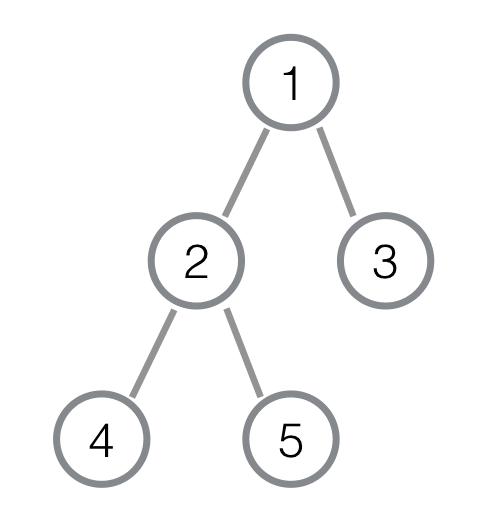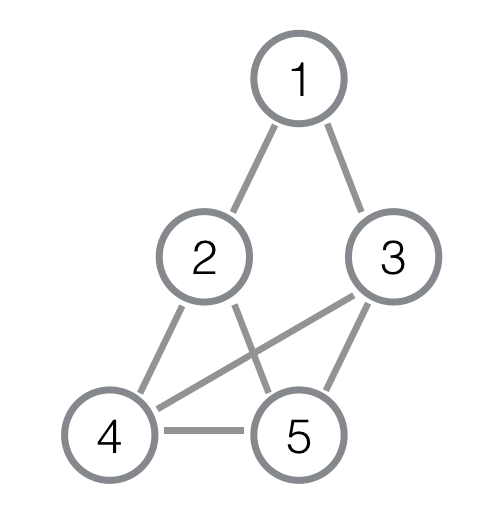# #398. 【NOI2018】多边形• 从根节点开始深度优先遍历整棵树，遍历时按照编号从小到大的顺序来访问孩子，这样可以得到一个树 $T$ 的 DFS 序。
• 接着按照在 DFS 序中的出现顺序从前往后，久莲把所有叶子节点排成一排得到了一个序列 $A$。

• 在树 $T$ 中存在连接 $u, v$ 的边。
• 在树 $T$ 中 $u, v$ 都是叶子节点且 $d(u, v) \le K$。• 无重边无自环的无向图 $G$ 的一条哈密尔顿回路 $H$ 是 $G$ 中边的一个子集，其中每一个点恰好有两条不同的相邻边在 $H$ 中，且任意两个点都可以通过 $H$ 中的边直接或间接到达。
• 无重边无自环的无向图 $G$ 的两条哈密尔顿回路 $H_1, H_2$ 是不同的当且仅当存在一条边 $e$ 使得 $e$ 在 $H_1$ 中且不在 $H_2$ 中。
• DFS 序是从 $1$ 号点开始对整棵树进行深度优先遍历时被访问节点按照从先到后的顺序形成的序列。

### 样例一

#### input

5 1
1 1 2 2



#### output

2



### 限制与约定

$1$$\le 5$$\le 3$$11$$\le 1000$$\le 2A 2$$\le 10$$\le 3$$12$$\le 1000$$\le 2$A
$3$$\le 15$$\le 3$$13$$\le 1000$$\le 2A 4$$\le 20$$\le 3$$14$$\le 1000$$\le 2$
$5$$\le 1000$$=1$A$15$$\le 1000$$\le 2$
$6$$\le 1000$$=1$A$16$$\le 1000$$\le 2$
$7$$\le 1000$$=1$A$17$$\le 1000$$\le 3$A
$8$$\le 1000$$=1$$18$$\le 1000$$\le 3A 9$$\le 1000$$=1$$19$$\le 1000$$\le 3$
$10$$\le 1000$$=1$$20$$\le 1000$$\le 3$